# Less Than

Less Than

If you ever wondered how to identify where to put the less-than symbol or the greater than symbol, think of the alligator.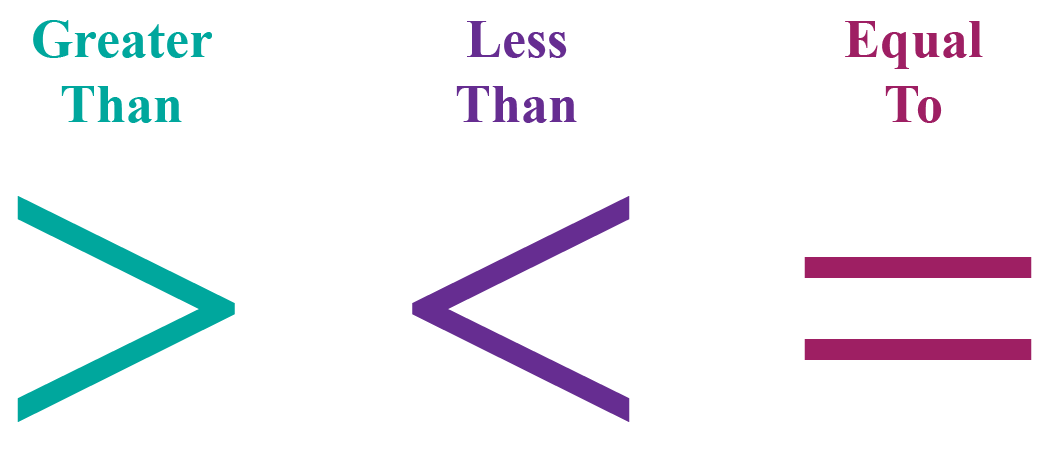The alligator’s mouth will open in the direction of the larger food item, in this case, a number. Once you get a hang of the symbols, you will understand numbers and fractions that are less than 1, as well as the concept of less than or equal to. Check-out the interactive simulations to know more about the lesson and try your hand at solving a few interesting practice questions at the end of the page.

## Lesson Plan

 1 What is Less Than? 2 Solved Examples on Less Than 3 Interactive Questions on Less Than

## What Is Less Than?

In mathematics, we use numbers for comparisons.

To compare means examining the difference between quantities or values to decide which is greater than, or equal to, or smaller than the other quantity.

We do comparisons in terms of weight, the volume of liquids, etc.

In mathematics, we have important symbols or signs to understand bigger numbers, smaller numbers, and equal numbers.

We use certain signs for comparing these numbers, and "less than" is one such sign.

One of the ways to compare numbers is by using a number line.

Let's try the simulation shown below.

Enter any two numbers between -4 and 8

You can then observe them on the number line along with the symbol showing their relationship.

## Define Less Than

Less than is used to describe the relationship between two values.

Less than meaning: Less than indicates that one value is lesser than the other.

The sign for less than is <

Using this symbol, we can compare numbers, weights, distances, etc.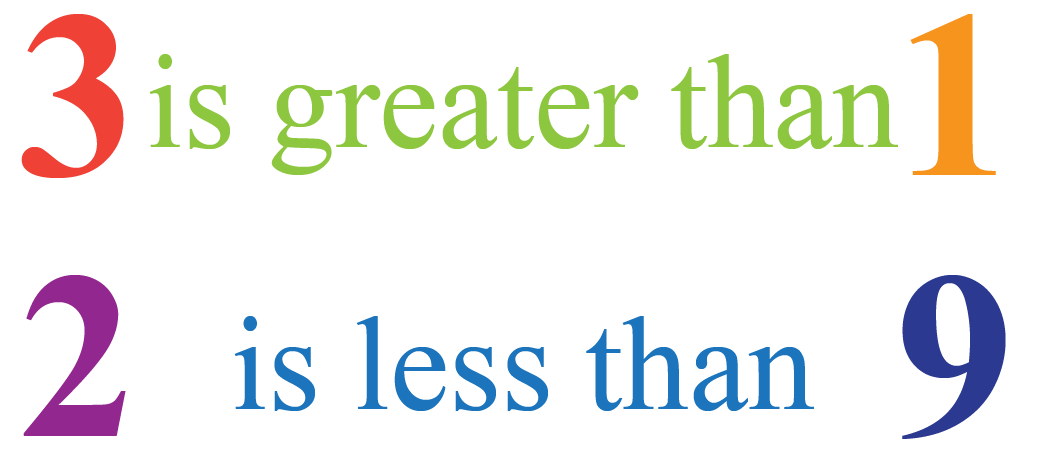We can show these numbers as: 3 > 1, 2 < 9Think Tank

1. Write the following statement using mathematical symbols.

a) 5 is less than the sum of 3 and 4

b) The product of 8 and 2 is equal to the product of 2 and 8

## What Is the Symbol of Less Than?

This sign is called the less than sign in math.This is used to show that one value is lesser than the other value.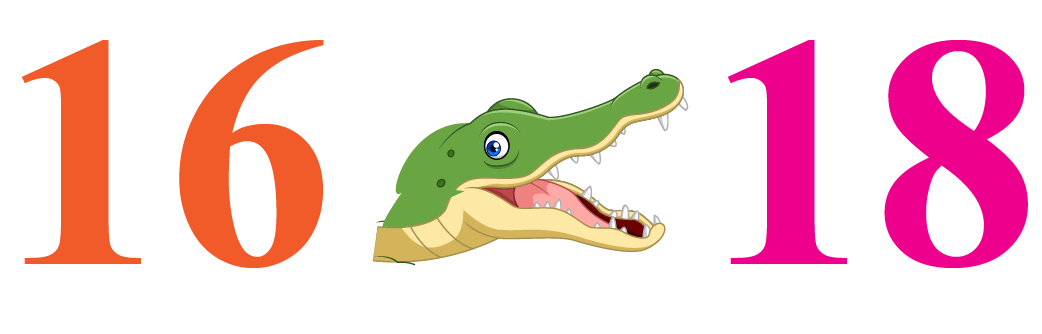We know that the statement "16 is less than 18" is true.

Hence, we can write it as 16 < 18 using the less than symbol.

The wide-open side of the sign with its two points always faces the bigger number, whereas the smaller endpoint faces the smaller number.Tips and Tricks
1. One of the easiest ways to remember the "greater than" and "less than" signs is to imagine them as hungry alligators that always eat the larger number.

Hence, the wide-open side of the sign faces the larger number.

2.  Another way to memorize them is to observe that the sign "<" looks similar to the alphabet "L". In other words, "<" means "Less than."

## When to use Less Than?

Let's compare the two-digit numbers, 32 and 14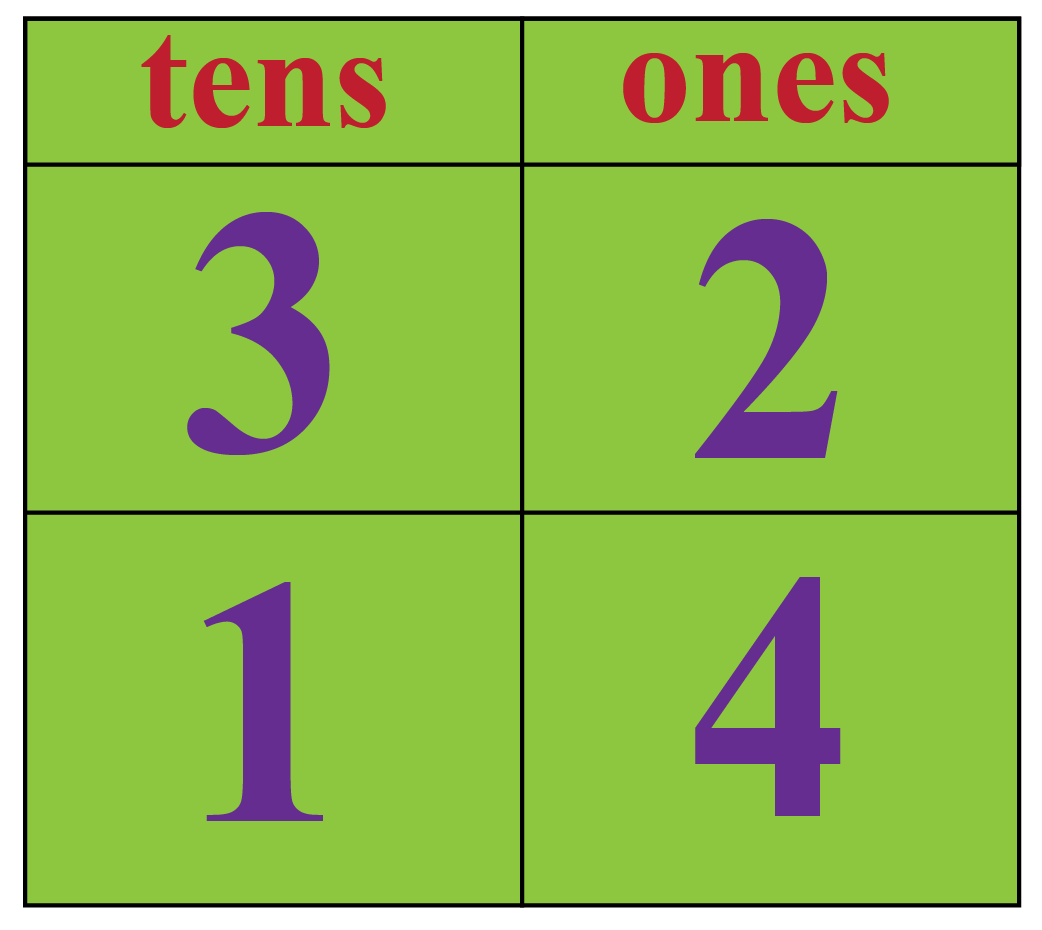While comparing the two-digit numbers, we first compare the tens digits.

If the tens digits are the same, we will compare the ones digits.

Here, we can see that 1 is less than 3

Thus, we can say that 14 is less than 32

Mathematically, we can write it as 14 < 32 using the less than symbol.

### Less Than 1

Representing numbers on a number line makes it easy to identify which numbers are greater or lesser.

A number on the left is less than a number on the right.

Hence, all the numbers which are on the left of 1 are less than 1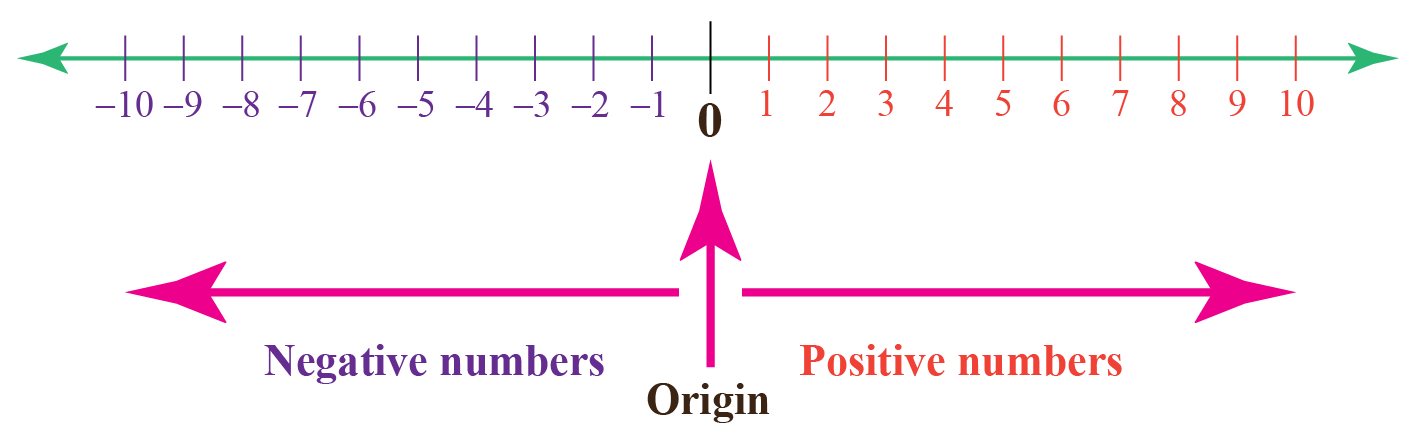## Use of Less Than in Algebra

In mathematics, the less than and the greater than symbols describe the inequality between two values.

The symbol  “< “ is used to represent less than inequality.Challenging Questions
1. Make the smallest four-digit number using the digits: 7, 1, 4, and 2 in such a way that no digit is repeated.
2. For the numbers 0.12 and 0.21, form a mathematical statement using "less than symbol".

## Solved Examples

 Example 1

Observe the figure below.

On the right side, there are 6 stars.

On the left side, there are 4 stars.

Insert the appropriate sign between the numbers.Solution

The number 4 is smaller than the number 6

Hence, the alligator's open mouth faces number 6Here, we can say that 4 < 6

 $$\therefore$$  4 < 6
 Example 2

Amrita had to collect various kinds of leaves and paste them in 2 sheets.

In order to place them all in two sheets, she pasted 8 on one sheet and 9 on the other.

Which sheet has fewer leaves?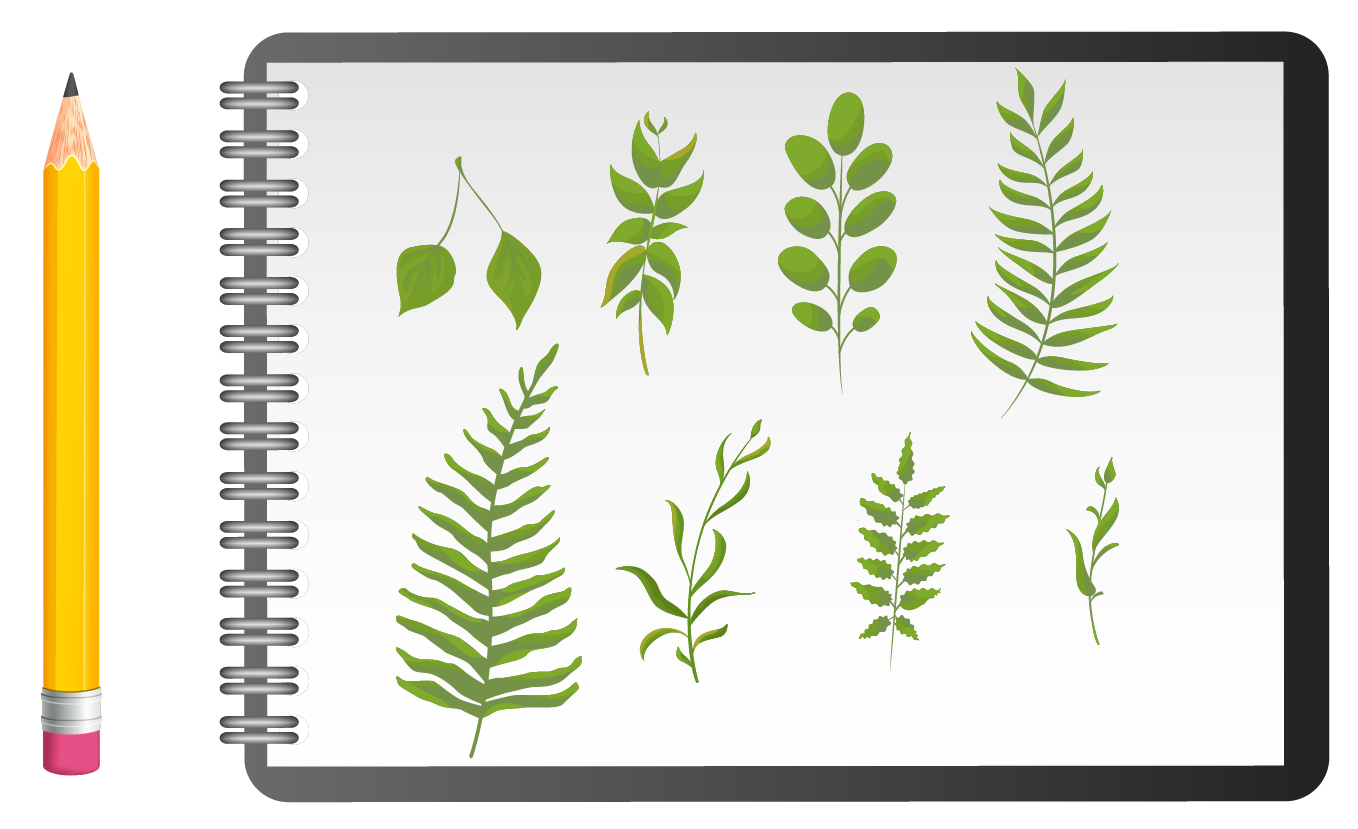Solution

As a part of her assignment, Amrita pasted 8 leaves on one page and 9 on the other.

The number 8 is less than the number 9

Thus, the sheet with 8 leaves has fewer leaves.

 $$\therefore$$  The sheet with 8 leaves has fewer leaves.
 Example 3

A cow weighs 720 kilogram.

The weight of her calf is 180 kilogram.

Which of them weighs less?

Use symbols to represent this information.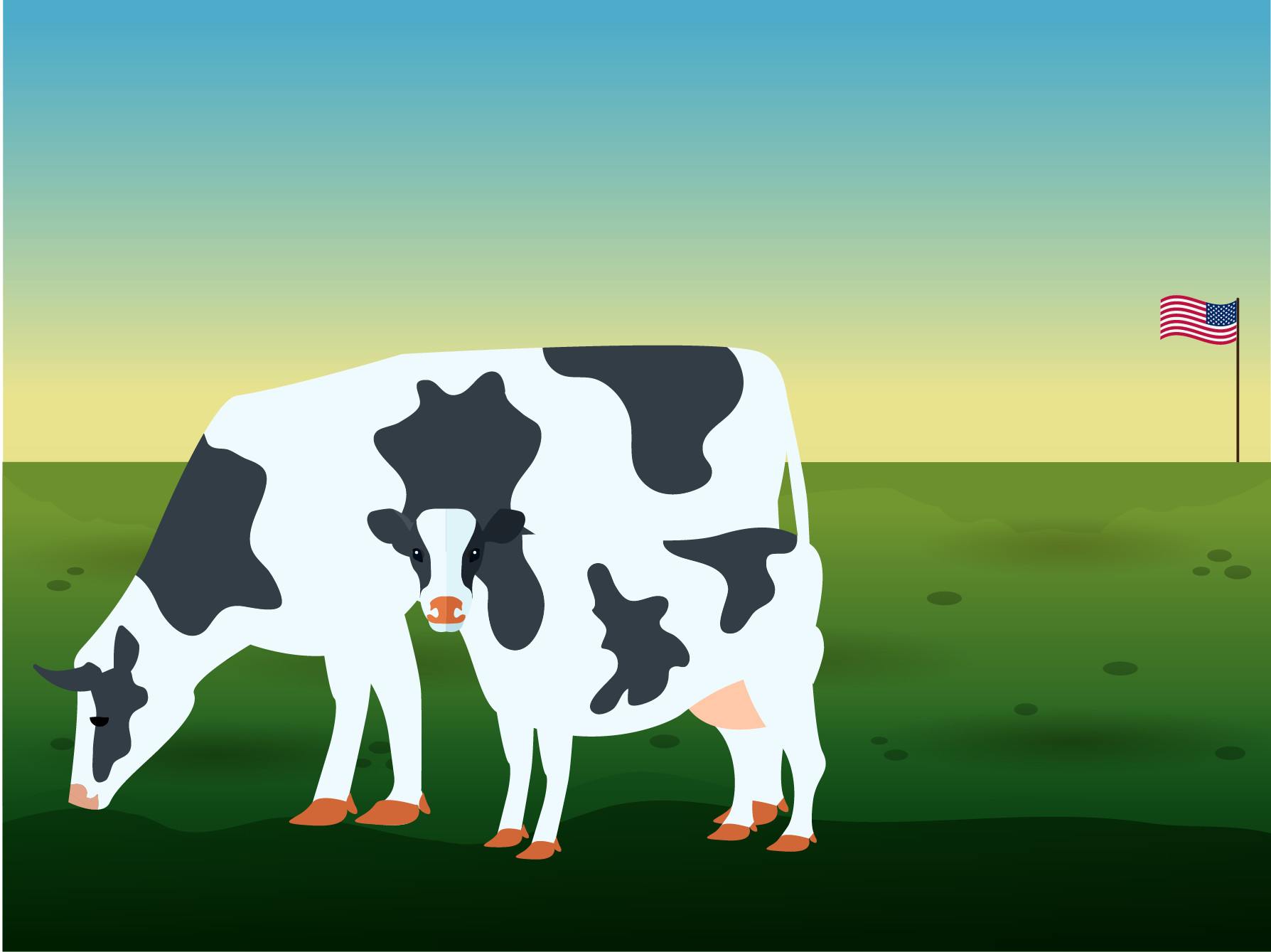Solution

Weight of the cow = 720 kilogram

Weight of the calf = 180 kilogram

Let's observe the numbers, 720 and 180

They are three-digit numbers.

7 and 1 are at the hundredths place.

By comparing them, we can say that 1 is less than 7

Thus, the information is represented as 180 < 720

 $$\therefore$$  The information is represented as 180 < 720
 Example 4

(i) Which of them weighs less?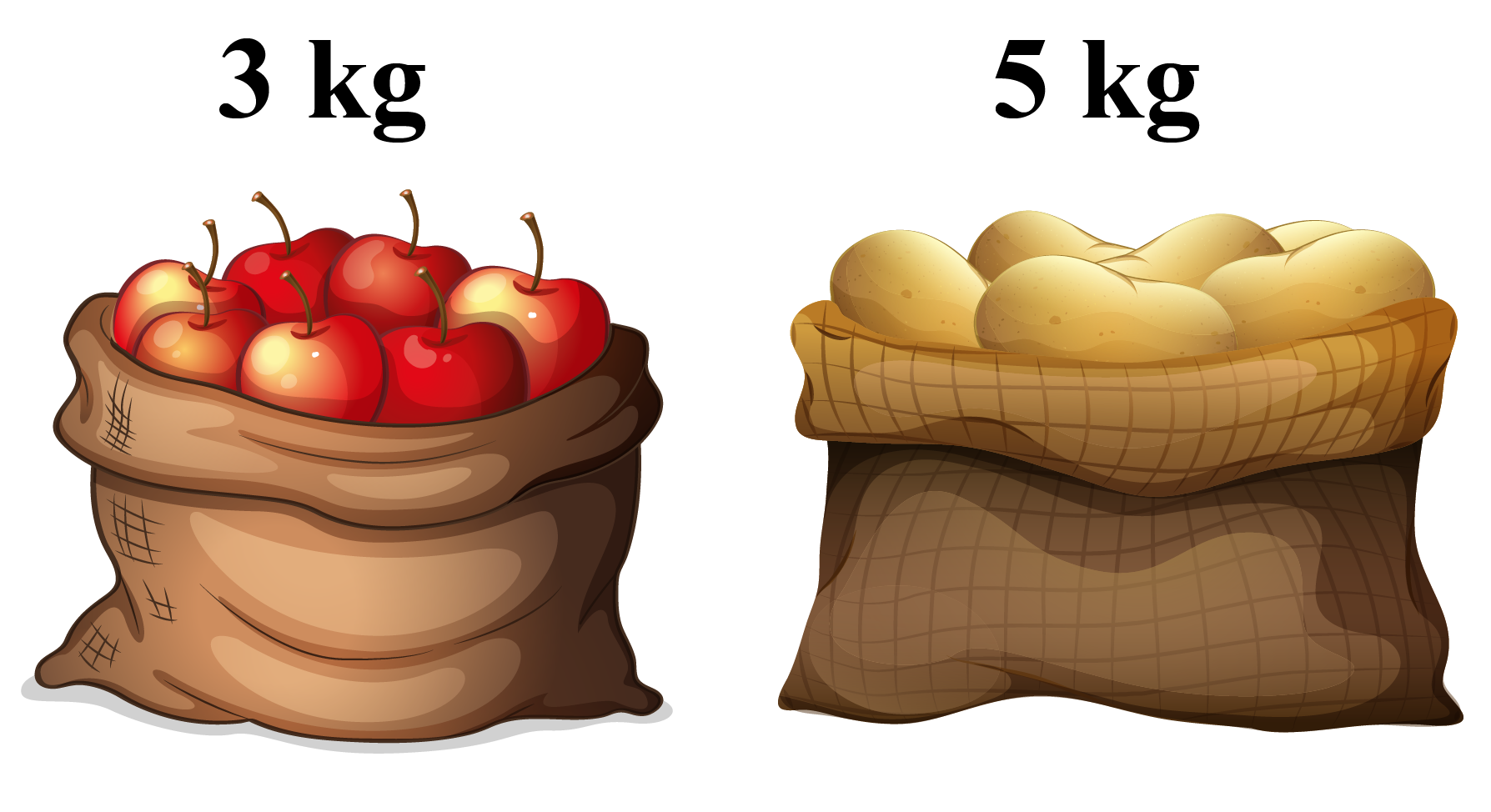(a)                                                              (b)

(ii) Which of them (the bottle or the cartons) holds less amount of liquid?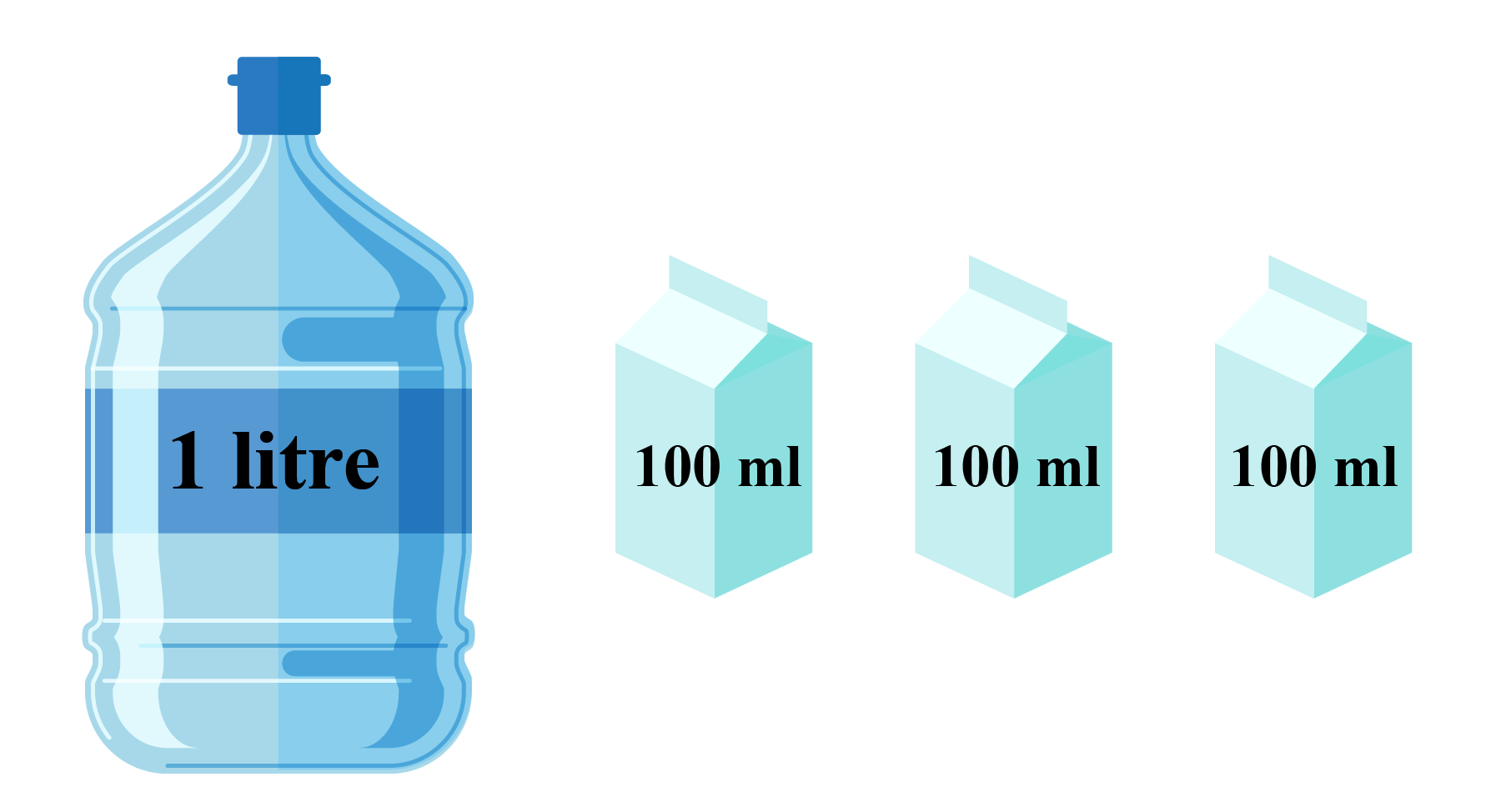Solution

 $$\therefore$$ (i) (a) weighs less. (ii) The carton each of capacity 100 ml holds less than the 1 litre(1000 ml) bottle.
 Example 5

Varun is working on an assignment for his class.

He has a list of states which need to be arranged in ascending order according to their 2016 wheat harvest.

 States Volume (in million tonnes) Uttar Pradesh 6812 Haryana 5805 Punjab 6206

Can you help him complete this task?

Solution

Arranging the numbers in ascending order means to arrange them from the lowest to the highest.

You can notice that all these numbers are not single-digit numbers.

Hence, we will start comparing from the left-most digit in each number.

Thus, we get, 5805 < 6206 < 6812

That is,  5805 million tonnes < 6206 million tonnes < 6812 million tonnes.

 $$\therefore$$  5805 million tonnes < 6206 million tonnes < 6812 million tonnes

## Interactive Questions

Here are a few activities for you to practice.

Select/Type your answer and click the "Check Answer" button to see the result.

## Let's Summarize

We hope you enjoyed learning about Less Than with the simulations and practice questions. Now you will be able to easily solve problems on less than symbol, less than meaning, less than or equal to symbol in words, and less than 1

## About Cuemath

At Cuemath, our team of math experts is dedicated to making learning fun for our favorite readers, the students!

Through an interactive and engaging learning-teaching-learning approach, the teachers explore all angles of a topic.

Be it worksheets, online classes, doubt sessions, or any other form of relation, it’s the logical thinking and smart learning approach that we, at Cuemath, believe in.

## 1.  Are 0.5 and 0.05 the same?

No, 0.5, and 0.05 are not the same. They are not equal.

0.05 = $$\dfrac{5}{100}$$ and 0.5= $$\dfrac{5}{10}$$

0.05 = $$\dfrac{5}{100}$$ < 0.5= $$\dfrac{50}{100}$$

0.05 < 0.5

## 2. Can less than be represented on a number line?

Yes, less than can be represented on the number line.

## 3. What number is less than a negative number?

On the number line, negative numbers are to the left of zero.

The greatest negative integer is -1. There is no smallest negative integer.

More Important Topics
Numbers
Algebra
Geometry
Measurement
Money
Data
Trigonometry
Calculus